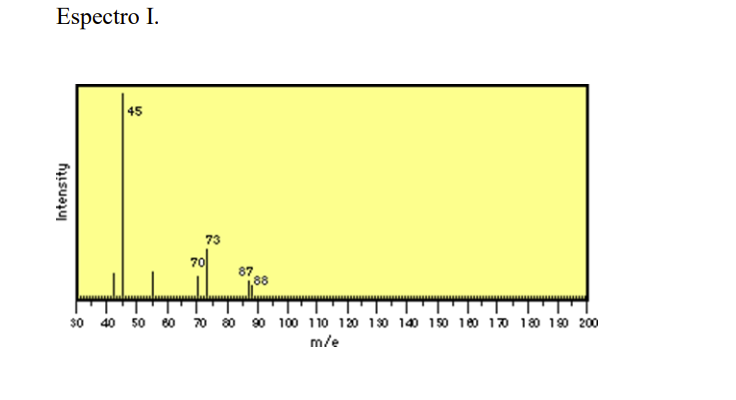# Question Below you will find several mass spectra, use the following concepts to explain: a) the molecular ion; b) rule thirteen to propose the basic formula; c) the rate of hydrogen deficiency or rate of establishment; d) explain the presence or absence of halogens; e) propose a formula for each spectrum; f) propose the structure of the fragment which produces the base peak. Espectro I.Below you will find several mass spectra, use the following concepts to
explain: a) the molecular ion; b) rule thirteen to propose the basic formula; c) the rate of
hydrogen deficiency or rate of establishment; d) explain the presence or absence of
halogens; e) propose a formula for each spectrum; f) propose the structure of the fragment
which produces the base peak.Transcribed Image Text: Espectro I.
More
Transcribed Image Text: Espectro I.